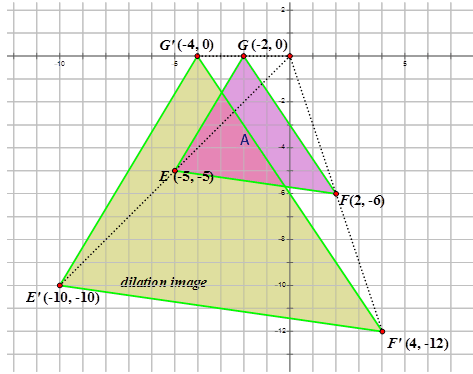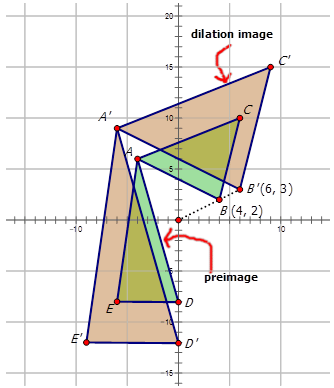# 7.17: Mapping Dilations

•• Contributed by CK12
• CK12

Figures that can be carried to each other using one or more rigid transformations followed by a dilation. Rules that describe given size changes of images

## Rules for Dilations

The figure below shows a dilation of two trapezoids. Write the mapping rule for the dilation of Image A to Image B.Figure $$\PageIndex{1}$$

In geometry, a transformation is an operation that moves, flips, or changes a shape to create a new shape. A dilation is a type of transformation that enlarges or reduces a figure (called the preimage) to create a new figure (called the image). The scale factor, $$r$$, determines how much bigger or smaller the dilation image will be compared to the preimage.

Look at the diagram below:Figure $$\PageIndex{2}$$

The Image A has undergone a dilation about the origin with a scale factor of 2. Notice that the points in the dilation image are all double the coordinate points in the preimage. A dilation with a scale factor $$k$$ about the origin can be described using the following notation:

$$D_{k} (x,y)=(kx,ky)$$

$$k$$ will always be a value that is greater than 0.

Scale Factor, k Size change for preimage
$$k>1$$ Dilation image is larger than preimage
$$0<k<1$$ Dilation image is smaller than preimage
$$k=1$$

Dilation image is the same size as the preimage

#### Let's draw the dilation image for the following mappings:

1. The mapping rule for the dilation applied to the triangle below is $$(x,y)\rightarrow (1.5x,1.5y)$$.Figure $$\PageIndex{3}$$

With a scale factor of 1.5, each coordinate point will be multiplied by 1.5.

$$\begin{array}{llcc} \text { Image } A & A(3,5) & B(4,2) & C(1,1) \\ \text { Dilation Image } & A^{\prime}(4.5,7.5) & B^{\prime}(6,3) & C^{\prime}(1.5,1.5) \end{array}$$

The dilation image looks like the following:Figure $$\PageIndex{4}$$
1. The mapping rule for the dilation applied to the diagram below is $$(x,y)\rightarrow (\dfrac{1}{3}x, \dfrac{1}{3}y)$$.Figure $$\PageIndex{5}$$

With a scale factor of $$\dfrac{1}{3}$$, each coordinate point will be multiplied by $$\dfrac{1}{3}$$.

$$\begin{array}{llllr} \text { Image } D & D(-3,7) & E(-1,3) & F(-7,5) & G(-5,1) \\ \text { Dilation Image } & D^{\prime}(-1,2.3) & E^{\prime}(-0.3,1) & F^{\prime}(-2.3,1.7) & G^{\prime}(-1.7,0.3) \end{array}$$

The dilation image looks like the following:Figure $$\PageIndex{6}$$

Now, let's write the notation that represents the dilation of the preimage A to the dilation image J in the diagram below:Figure $$\PageIndex{7}$$

First, pick a point in the diagram to use to see how it has been affected by the dilation.

$$C:(−7,5)\qquad C′:(−1.75,1.25)$$

Notice how both the x- and y-coordinates are multiplied by $$\dfrac{1}{4}$$. This indicates that the preimage $$A$$ undergoes a dilation about the origin by a scale factor of $$\dfrac{1}{4}$$ to form the dilation image $$J$$. Therefore the mapping notation is $$(x,y)\rightarrow (\dfrac{1}{4}x,\dfrac{1}{4}y)$$.

Example $$\PageIndex{1}$$

Earlier, you were asked to write the mapping rule for the dilation of Image A to Image B in the image below:Figure $$\PageIndex{8}$$

Solution

Look at the points in each image:

$$\begin{array}{lllll} \text { Image } A & B(-9,6) & C(-5,6) & D(-5,-1) & E(-10,-3) \\ \text { Image } B & B^{\prime}(-4.5,3) & C^{\prime}(-2.5,3) & D^{\prime}(-2.5,-0.5) & E^{\prime}(-5,-1.5) \end{array}$$

Notice that the coordinate points in Image B (the dilation image) are \dfrac{1}{2}\) that found in Image A\). Therefore the Image A undergoes a dilation about the origin of scale factor \dfrac{1}{2}\). To write a mapping rule for this dilation you would write: (x,y)\rightarrow (\dfrac{1}{2}x,\dfrac{1}{2}y)\).

Example $$\PageIndex{2}$$

Thomas describes a dilation of point $$JT$$ with vertices $$J(−2,6)$$ to $$T(6,2)$$ to point $$J′T′$$ with vertices $$J′(−4,12)$$ and $$T′(12,4)$$. Write the notation to describe this dilation for Thomas.Figure $$\PageIndex{9}$$

Solution

Since the x- and y-coordinates are each multiplied by 2, the scale factor is 2. The mapping notation is: $$(x,y)\rightarrow (2x,2y)$$

Example $$\PageIndex{3}$$

Given the points $$A(12,8)$$ and $$B(8,4)$$ on a line undergoing a dilation to produce $$A′(6,4)$$ and $$B′(4,2)$$, write the notation that represents the dilation.

Solution

In order to write the notation to describe the dilation, choose one point on the preimage and then the corresponding point on the dilation image to see how the point has moved. Notice that point EA\) is:

$$A(12,8)\rightarrow A′(6,4)$$

Since both x- and y-coordinates are multiplied by $$\dfrac{1}{2}$$, the dilation is about the origin has a scale factor of $$\dfrac{1}{2}$$. The notation for this dilation would be: $$(x,y)\rightarrow (\dfrac{1}{2}x,\dfrac{1}{2}y)$$.

Example $$\PageIndex{4}$$

Janet was playing around with a drawing program on her computer. She created the following diagrams and then wanted to determine the transformations. Write the notation rule that represents the transformation of the purple and blue diagram to the orange and blue diagram.Figure $$\PageIndex{10}$$

Solution

In order to write the notation to describe the dilation, choose one point on the preimage $$A$$ and then the corresponding point on the dilation image $$A′$$ to see how the point has changed. Notice that point $$E$$ is shown in the diagram:

$$E(−5,−3)\rightarrow E′(−1,−0.6)$$

Since both x- and y-coordinates are multiplied by $$\dfrac{1}{5}$$, the dilation is about the origin has a scale factor of $$\dfrac{1}{5}$$. The notation for this dilation would be: $$(x,y)\rightarrow (\dfrac{1}{5}x, \dfrac{1}{5}y)$$.

## Review

Complete the following table. Assume that the center of dilation is the origin.

Starting Point $$D_{2}$$ $$D_{5}$$ $$D_{\dfrac{1}{2}}$$ $$D_{\dfrac{3}{4}}$$
1. (1, 4)
2. (4, 2)
3. (2, 0)
4. (-1, 2)
5. (-2, -3)
6. (9, 4)
7. (-1, 3)
8. (-5, 2)
9. (2, 6)
10. (-5, 7)

Write the notation that represents the dilation of the preimage to the image for each diagram below.

1.Figure $$\PageIndex{11}$$
2.Figure $$\PageIndex{12}$$
3.Figure $$\PageIndex{13}$$
4.Figure $$\PageIndex{14}$$
5.Figure $$\PageIndex{15}$$